# Test: Monotonicity (Competition Level) - 1

## 22 Questions MCQ Test Mathematics (Maths) Class 12 | Test: Monotonicity (Competition Level) - 1

Description
This mock test of Test: Monotonicity (Competition Level) - 1 for JEE helps you for every JEE entrance exam. This contains 22 Multiple Choice Questions for JEE Test: Monotonicity (Competition Level) - 1 (mcq) to study with solutions a complete question bank. The solved questions answers in this Test: Monotonicity (Competition Level) - 1 quiz give you a good mix of easy questions and tough questions. JEE students definitely take this Test: Monotonicity (Competition Level) - 1 exercise for a better result in the exam. You can find other Test: Monotonicity (Competition Level) - 1 extra questions, long questions & short questions for JEE on EduRev as well by searching above.
QUESTION: 1

Solution:
QUESTION: 2

### The function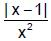is monotonically decreasing in

Solution:

f(x)= {−(x−1)/x2   x<1,   x(x−1)/x2  x≥1}
​f'(x)= {(x−2)/x3   x<1,      −(x−2)/x3   x≥1}
​x<1, if f′(x)<0 (for f(x) to be monotonically decreasing
⇒ (x−2)/x3<0
⇒x∈(0,2)
But x<1 ⇒ x∈(0,1)
For x≥1, if f′(x)<0
⇒ −(x−2)]/x3<0
⇒(x−2)/x3 > 0
⇒x∈(−∞,0)∪(2,∞)
But, x≥1   ⇒x∈(2,∞)
Hence, x∈(0,1)∪(2,∞)

QUESTION: 3

### If y = (a + 2) x3 – 3ax2 + 9ax – 1 decreases monotonically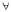x ∈ R then `a' lies in the interval

Solution:
QUESTION: 4

The true set of real values of x for which the function, f(x) = x ln x – x + 1 is positive is

Solution:

f(x)=xlnx−x+1
f(1)=0
On differentiating w.r.t x, we get
f′(x)=x*1/x+lnx−1
=lnx
Therefore,f′(x)>0 for allx∈(1,∞)
f′(x)<0 for allx∈(0,1)
lim x→0 f(x)=1
f(x)>0
for allx∈(0,1)∪(1,∞)

QUESTION: 5

The set of all x for which ln (1 + x) ≤ x is equal to

Solution:

f(x) = ln(1+x) - x ≤ 0
f(x) = ln(1+x) - x
f’(x) = 1/(1+x) - 1
= (1 - 1 - x)/(1+x)
= -x/(1+x)
f’(x) ≤ 0
-x/(1+x) ≤ 0
0 ≤ x /(1+x)
for(x = 2) (-2)/(1-2)
= 2 > 0, therefore x > - 1

QUESTION: 6

The curve y = f(x) which satisfies the condition f'(x) > 0 and f"(x) < 0 for all real x, is

Solution:

f’(x) > 0
=> f(x) is increasing
f’’(x) < 0  => f(x) is convex

QUESTION: 7

If the point (1, 3) serves as the point of inflection of the curve y = ax3 + bx2 then the value of `a' and `b' are

Solution:
QUESTION: 8

The function f(x) = x3 – 6x2 + ax + b satisfy the conditions of Rolle's theorem in [1, 3]. The value of a and b are

Solution:
QUESTION: 9

If f(x) =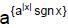; g(x) =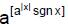for a > 1, a ¹ 1 and x Î R, where {*} & [*] denote the fractional part and integral part functions respectively, then which of the following statements holds good for the function h(x), where (ln a) h(x) = (ln f(x) +ln g(x)).

Solution:
QUESTION: 10

Let f(x) = (x – 4) (x – 5) (x – 6) (x – 7) then,

Solution:
QUESTION: 11

Given that f is a real valued differentiable function such that f(x) f'(x) < 0 for all real x, it follows that

Solution:
QUESTION: 12

If f(x) =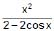; g(x) =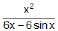where 0 < x < 1, then

Solution:
QUESTION: 13

f : R → R be a differentiable function x ∈ R. If tangent drawn to the curve at any point x ∈ (a, b) always lie below the curve, then

Solution:
QUESTION: 14

A value of C for which the conclusion of Mean Value Theorem holds for the function f(x) = loge x on the interval [1, 3] is

Solution:
QUESTION: 15

The function f(x) = x(x + 3) e–x/2 satisfies all the conditions of Rolle’s theorem in [–3, 0]. The value of c which verifies Rolle’s theorem, is

Solution:

f(x) = x (x + 3)e–x/2 is continuous in [–3, 0]
and f′(x)=(x2 + 3x) (– 1/2)e-x/2 + (2x + 3)e–x/2
= – 1/2(x2 – x – 6)e–x/2
Therefore f′(x) exists (i.e., finite) for all x
Also f(–3) = 0, f (0) = 0
So that f(–3) = f(0)
Hence all the three conditions of the theorem are satisfied.
Now consider f′(c)=0
i.e., –1/2(c2 – c – 6)e–c/2 = 0
c2 – c – 6 = 0
(c + 2) (c – 3) = 0 c = 3 or –2
Hence there exists – 2 ∈ (–3, 0) such that
f′(–2) = 0

QUESTION: 16

x3 – 3x2 – 9x + 20 is

Solution:
QUESTION: 17

f(x) = x2 - x sin x is

Solution:
QUESTION: 18

The number of values of `c' of Lagrange's mean value theorem for the function,

f(x) = (x – 1) (x – 2) (x – 3), x ∈ (0, 4) is

Solution:
QUESTION: 19

If f(x) and g(x) are differentiable in [0, 1] such that f(0) = 2, g(0) = 0, f(1) = 6, g(1) = 2, then Rolle's theorem is applicable for which of the following

Solution:
QUESTION: 20

Equation 3x2 + 4ax + b = 0 has at least one root in (0, 1) if

Solution:
QUESTION: 21

Function for which LMVT is applicable but Rolle's theorem is not

Solution:
QUESTION: 22

LMVT is not applicable for which of the following ?

Solution: